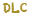When clearing a room, the game has a chance of rewarding a pickup or trinket. This is based on many things, including your current luck and whether you have some particular items.

## Process

First, the game generates a random number using this formula:

(RandomFloat * Luck * 0.1) + pickupPercent

• RandomFloat and pickupPercent are random numbers between 0 and 1
• Luck is clamped to 0 if Isaac’s luck is less than 0 and 10 if Isaac’s luck is greater than 10
• This chance is modified by the following items/trinkets:
• multiplies RandomFloat and pickupPercent by 0.9, then adds 0.1 to both of them.
• multiplies pickupPercent by 0.98, then adds 0.02 to it.

That number is then used to determine the reward given after clearing the room, using the following index:

• Nothing (< 0.22, base 22% chance)
• A tarot card, pill, or trinket (0.22 - 0.3, base 8% chance)
• All three possibilities are equally likely to be chosen, giving them individual base 2.66% chances.
• A coin (0.3 - 0.45, base 15% chance)
• The range increases to 0.3 - 0.5 (base 20%) if Isaac has• A heart (0.45 - 0.6, base 15% chance)
• The range decreases to 0.5 - 0.6 (base 10%) if Isaac has Rib of Greed
• If Isaac has , the heart has an 80% chance of being replaced with a key.
• A key (0.6 - 0.8, base 20% chance)
• A bomb (0.8 - 0.95, base 15% chance)
• A chest (> 0.95, base 5% chance)
• If Isaac has positive luck, the chance for a chest increases and all other chances decrease.
• Lucky Foot/Toe decrease the chance of getting nothing, but do not themselves increase the chance for chests to spawn.

The reward then has a chance to be replaced/modified by the following, in order:

• A lil' battery (3.33% chance if Isaac has)
• A sack (2% chance, always in effect)

• A tarot card (10% chance if Isaac has )
• A pill (10% chance if Isaac has )
• A bomb (10% chance if Isaac has )
• A heart (10% chance if Isaac has )
• A key (10% chance if Isaac has )
• If Isaac has multiple of these, the first one to activate is the one that replaces the room reward
• A trinket (2% chance if Isaac has)
• has a 33% chance to replace the reward with a chest (either gray or locked with equal chances) and a 33% chance to replace it with nothing.
• causes an extra copy of the reward to spawn, but has a 33% chance to replace it with nothing.
• Each extra contract Isaac has causes another copy of the reward to spawn and halves the chance the reward becomes nothing.
• If playing on Hard Mode, heart rewards have a66%/33% chance to be replaced with nothing.
•has a 25% chance to create an additional copy of the reward if it’s a key, coin, heart, bomb, or sack.

## Pseudo-code

The full logic is contained within the following pseudo-code:

```local room = Game():GetLevel():GetCurrentRoom()
local awardSeed = room.AwardSeed
local player = Game():GetPlayer(0)
local difficulty = Game().Difficulty

local rng = RNG()
rng:SetSeed(awardSeed, 35) --5, 9, 7

local pickupPercent = rng:RandomFloat()
if (player:HasCollectible(COLLECTIBLE_LUCKYFOOT)) then
pickupPercent = (pickupPercent * 0.9) + 0.1
end

local luck = math.max(math.min(player.Luck, 10), 0) --Clamp to 0-10

--Max luck increases the pickupPercent range from 0-1 to 0-2
--That means the more luck you have, the more likely you are to get chests.
pickupPercent = rng:RandomFloat() * luck * 0.1 + pickupPercent

if (player:HasTrinket(TRINKET_LUCKY_TOE)) then
if (player:HasCollectible(COLLECTIBLE_LUCKYFOOT) and luck > 0) then
pickupPercent = (pickupPercent * 0.98) + 0.02
else
pickupPercent = (pickupPercent * 0.9) + 0.1
end
end

local pickupAward = COLLECTIBLE_NULL
local pickupCount = 1

if (pickupPercent > 0.22) then
if (pickupPercent < 0.3) then
if (rng:RandomInt(3) == 0) then
pickupAward = PICKUP_TAROTCARD
elseif (rng:RandomInt(2) == 0) then
pickupAward = PICKUP_TRINKET
else
pickupAward = PICKUP_PILL
end
elseif (pickupPercent < 0.45) then
pickupAward = PICKUP_COIN
elseif (pickupPercent < 0.5 and player:HasTrinket(TRINKET_RIB_OF_GREED)) then
pickupAward = PICKUP_COIN
elseif (pickupPercent < 0.6 and (not player:HasTrinket(TRINKET_DAEMONS_TAIL) or rng:RandomInt(5) == 0)) then
pickupAward = PICKUP_HEART
elseif (pickupPercent < 0.8) then
pickupAward = PICKUP_KEY
elseif (pickupPercent < 0.95) then
pickupAward = PICKUP_BOMB
else
pickupAward = PICKUP_CHEST
end

if (rng:RandomInt(20) == 0 or (rng:RandomInt(15) == 0 and player:HasTrinket(TRINKET_WATCH_BATTERY))) then
pickupAward = PICKUP_LIL_BATTERY
end

if (rng:RandomInt(50) == 0) then
pickupAward = PICKUP_GRAB_BAG
end

if (player:HasTrinket(TRINKET_ACE_SPADES) and rng:RandomInt(10) == 0) then
pickupAward = PICKUP_TAROTCARD
elseif (player:HasTrinket(TRINKET_SAFETY_CAP) and rng:RandomInt(10) == 0) then
pickupAward = PICKUP_PILL
elseif (player:HasTrinket(TRINKET_MATCH_STICK) and rng:RandomInt(10) == 0) then
pickupAward = PICKUP_BOMB
elseif (player:HasTrinket(TRINKET_CHILDS_HEART) and rng:RandomInt(10) == 0 and (not player:HasTrinket(TRINKET_DAEMONS_TAIL) or rng:RandomInt(5) == 0)) then
pickupAward = PICKUP_HEART
elseif (player:HasTrinket(TRINKET_RUSTED_KEY) and rng:RandomInt(10) == 0) then
pickupAward = PICKUP_KEY
end

if (player:HasCollectible(COLLECTIBLE_SMELTER) and rng:RandomInt(50) == 0) then
pickupAward = PICKUP_TRINKET
end
end

if (player:HasCollectible(COLLECTIBLE_GUPPYS_TAIL)) then
if (rng:RandomInt(3) != 0) then
if (rng:RandomInt(3) == 0) then
pickupAward = PICKUP_NULL
end
else
if (rng:RandomInt(2) != 0) then
pickupAward = PICKUP_LOCKEDCHEST
else
pickupAward = PICKUP_CHEST
end
end
end

if (player:HasCollectible(COLLECTIBLE_CONTRACT_FROM_BELOW) and pickupAward != PICKUP_TRINKET) then
pickupCount = player:GetCollectibleNum(COLLECTIBLE_CONTRACT_FROM_BELOW) + 1
--The chance of getting nothing goes down with each contract exponentially
local nothingChance = math.pow(0.666, pickupCount - 1)
if (nothingChance * 0.5 > rng:NextFloat()) then
pickupCount = 0
end
end

if (difficulty == 1 and pickupAward == PICKUP_HEART) then
if rng:RandomInt(100) >= 35 then
pickupAward = PICKUP_NULL
end
end

if (player:HasCollectible(COLLECTIBLE_BROKEN_MODEM) and rng:RandomInt(4) == 0 and pickupCount >= 1 and
(pickupAward == PICKUP_COIN or pickupAward == PICKUP_HEART or pickupAward == PICKUP_KEY or pickupAward == PICKUP_GRAB_BAG or pickupAward == PICKUP_BOMB) then
pickupCount = pickupCount + 1
end

if (pickupCount > 0 and pickupAward != PICKUP_NULL) then
local subType = 0
for i=1, pickupCount do
local ent = Game():Spawn(ENTITY_PICKUP, pickupAward, nearCenter, Vector(0, 0), 0, subtype, rng:Next())
subType = ent.SubType
end
end

```